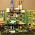# Max Frequency calculation

In the simplest form:
FF1 - combo - FF2 ( this is how things look physically for our consideration)
Tmin = Tclk2Q (FF1)+ Td(Comb0)+Tsu(FF2)

* mainly dependent on the critical path, and can do a good job by defining proper timing constraints during synthesis.

In detail:
Timing budget is the account of timing requirements or timing parameters necessary for a system to function properly. For synchronous systems to work, timing requirements must fit within one clock cycle. A timing-budget calculation involves many factors, including hold-time requirements and maximum operating frequency requirements. By calculating a timing budget, the limitations of conventional clocking methods can be seen.

Let's use an example for a system with standard clocking. Assume a memory controller interfacing with an SRAM. Both the SRAM and memory controller receive clock signals from the same clock source. It's assumed that clock traces are designed to match the trace delays. The relevant timing parameters are:
tSU (setup time) of memory controller
• tH (hold time) of memory controller
• tPD (propagation delay) of board trace
• tCO (clock to output delay) of SRAM
• tDOH (output data hold time) of SRAM
• tSKEW (clock skew) of clock generator
• tJIT (cycle-to-cycle jitter) of clock generator
• tCYC (cycle time) of clock generator

The maximum-frequency calculation gives the minimum cycle time of the system if the worst-case input setup time, clock to output time, propagation delay, clock skew, and clock jitter are considered.

The maximum frequency is given by:

tCO(max, SRAM) + tPD(max) + tSU(max, CTRL) + tSKEW(max, CLK) + tJIT(max, CLK)

The hold-time calculation verifies that the system outputs data too fast, violating input hold time of the receiving device in the system. In this case, the worst-case condition occurs when the data is driven out at the earliest possible time.

The formula is given by:

tCO(min, SRAM) + tPD(min) - tSKEW(min, CLK) - tJIT(min, CLK) > tH(max, CTRL)

Now let's assume the following values for the timing parameters of our SRAM and memory controller. In this case, we will use a high-speed SRAM with a double-data-rate (DDR) interface, where data is driven by the SRAM with every rising and falling edge of the clock.

tSU = 0.5 ns
tH = 0.4 ns
tCO = 0.45 ns
tDOH* = -0.45 ns
tSKEW = ±0.2 ns
tJIT = ±0.2 ns

*tDOH <>

The minimum hold-time requirement is calculated as:

tDOH + tPD - tSKEW - tJIT > tH
-0.45 ns + tPD - 0.2 ns - 0.2 ns > 0.4 ns
-0.85ns + tPD > 0.4 ns
tPD > 1.25 ns

Assuming that the delay per inch of an FR4 board trace is 160 ps/in., the trace length from SRAM to memory controller must be at least 7.82 in. Using 1.2 ns for tPD, the maximum operating frequency is calculated below. Because the SRAM has a DDR interface, the timing budget is based on a half cycle:

tCO + tPD + tSU + tSKEW + tJIT < tCYC/2 0.45 ns + 1.25 ns + 0.5 ns + 0.2 ns + 0.2 ns < tCYC/2 2.6 ns < tCYC/2 5.2 ns < tCYC 192 MHz > fCYC

With a 7.82-in. FR4 trace length and typical timing parameters, the timing budget requirements are met for an operating frequency of up to 192 MHz. In systems that have limited board space, the 7.82-in. minimum trace-length constraint becomes a difficult requirement to satisfy in systems.

If it isn't possible to introduce a trace delay, the memory controller can satisfy the hold-time requirement by using a delay-locked loop/phase-locked loop (DLL/PLL) to phase-shift the clock signal to capture data at an earlier time. The memory controller will have to resynchronize captured data with the system clock. Using this method will introduce additional PLL/DLL jitter, which decreases the system's maximum operating frequency. With the added delay of the PLL, the minimum hold-time requirement becomes:

tDOH + tPD(trace) + tPLL/DLL_DELAY - tSKEW - tJIT > tHtCO + tPD + tSU + tSKEW + tJIT
+ tJIT_PLL/DLL <>

Clock skew, clock jitter, and trace propagation delay can significantly limit system performance, even with the fastest SRAMs and ASICs/FPGAs available.

As mentioned earlier, the trace delay is approximately 160 ps/in. if an FR4 board is used. This is a significant number considering how the data-valid window at high frequencies has become 2 ns (e.g., for a 250-MHz, double-data-rate (DDR) device) and lower. Skew between the clock signals can also significantly reduce timing margins. We shall see that source-synchronous clocks can significantly reduce propagation delay, skew, and jitter, making timing closure more attainable.

1.Shouldn't maximum freq. be calculated by 1/time, not the addition of all the times?

2.Sorry for the confusion. It is 1/(total of all times).

3.Nice blog you got here. It would be great to read more concerning that topic. The only thing it would also be great to see on this blog is a photo of some blocker.

4.is tDOH always the negative of tco?

Nice blog post.

Thanks.